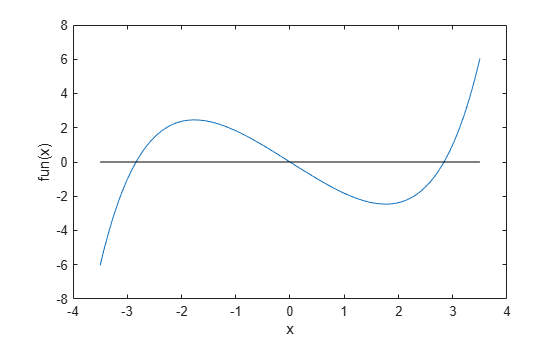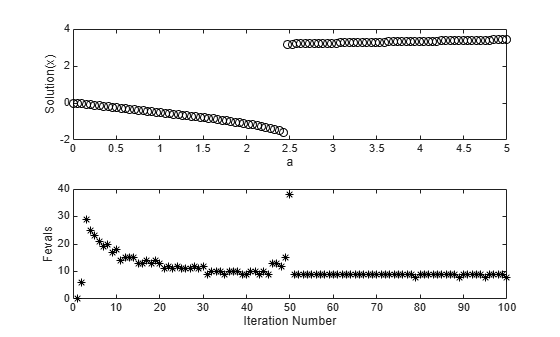# Follow Equation Solution as a Parameter Changes

This example shows how to solve an equation repeatedly as a parameter changes by starting subsequent solutions from the previous solution point. Often, this process leads to efficient solutions. However, a solution can sometimes disappear, requiring a start from a new point or points.

### Parameterized Scalar Equation

The parameterized equation to solve is

$\mathrm{sinh}\left(x\right)-3x=a$,

where $a$ is a numeric parameter that goes from 0 to 5. At $a=0$, one solution to this equation is $x=0$. When $a$ is not too large in absolute value, the equation has three solutions. To visualize the equation, create the left side of the equation as an anonymous function. Plot the function.

```fun = @(x)sinh(x) - 3*x; t = linspace(-3.5,3.5); plot(t,fun(t),t,zeros(size(t)),'k-') xlabel('x') ylabel('fun(x)')```When $a$ is too large or too small, there is only one solution.

### Problem-Based Setup

To create an objective function for the problem-based approach, create an optimization expression `expr` in an optimization variable `x`.

```x = optimvar('x'); expr = sinh(x) - 3*x;```

### Create and Plot Solutions

Starting from the initial solution $x=0$ at $a=0$, find solutions for 100 values of $a$ from 0 through 5. Because `fun` is a scalar nonlinear function, `solve` calls `fzero` as the solver.

Set up the problem object, options, and data structures for holding solution statistics.

```prob = eqnproblem; options = optimset('Display','off'); sols = zeros(100,1); fevals = sols; as = linspace(0,5);```

Solve the equation in a loop, starting from the first solution `sols(1) = 0`.

```for i = 2:length(as) x0.x = sols(i-1); % Start from previous solution prob.Equations = expr == as(i); [sol,~,~,output] = solve(prob,x0,'Options',options); sols(i) = sol.x; fevals(i) = output.funcCount; end```

Plot the solution as a function of the parameter `a` and the number of function evaluations taken to reach the solution.

```subplot(2,1,1) plot(as,sols,'ko') xlabel 'a' ylabel('Solution(x)') subplot(2,1,2) plot(fevals,'k*') xlabel('Iteration Number') ylabel('Fevals')```A jump in the solution occurs near $a=2.5$. At the same point, the number of function evaluations to reach a solution increases from near 15 to near 40. To understand why, examine a more detailed plot of the function. Plot the function and every seventh solution point.

```figure t = linspace(-3.5,3.5); plot(t,fun(t)); hold on plot([-3.5,min(sols)],[2.5,2.5],'k--') legend('fun','Maximum a','Location','north','autoupdate','off') for a0 = 7:7:100 plot(sols(a0),as(a0),'ro') if mod(a0,2) == 1 text(sols(a0) + 0.15,as(a0) + 0.15,num2str(a0/7)) else text(sols(a0) - 0.3,as(a0) + 0.05,num2str(a0/7)) end end plot(t,zeros(size(t)),'k-') hold off```As $a$ increases, at first the solutions move to the left. However, when $a$ is above 2.5, there is no longer a solution near the previous solution. `fzero` requires extra function evaluations to search for a solution, and finds a solution near `x = 3`. After that, the solution values move slowly to the right as $a$ increases further. The solver requires only about 10 function evaluations for each subsequent solution.

### Choose Different Solver

The `fsolve` solver can be more efficient than `fzero`. However, `fsolve` can become stuck in a local minimum and fail to solve the equation.

Set up the problem object, options, and data structures for holding solution statistics.

```probfsolve = eqnproblem; sols = zeros(100,1); fevals = sols; infeas = sols; asfsolve = linspace(0,5);```

Solve the equation in a loop, starting from the first solution `sols(1) = 0`.

```for i = 2:length(as) x0.x = sols(i-1); % Start from previous solution probfsolve.Equations = expr == asfsolve(i); [sol,fval,~,output] = solve(probfsolve,x0,'Options',options,'Solver','fsolve'); sols(i) = sol.x; fevals(i) = output.funcCount; infeas(i) = fval; end```

Plot the solution as a function of the parameter `a` and the number of function evaluations taken to reach the solution.

```subplot(2,1,1) plot(asfsolve,sols,'ko',asfsolve,infeas,'r-') xlabel 'a' legend('Solution','Error of Solution','Location','best') subplot(2,1,2) plot(fevals,'k*') xlabel('Iteration Number') ylabel('Fevals')````fsolve` is somewhat more efficient than `fzero`, requiring about 7 or 8 function evaluations per iteration. Again, when the solver finds no solution near the previous value, the solver requires many more function evaluations to search for a solution. This time, the search is unsuccessful. Subsequent iterations require few function evaluations for the most part, but fail to find a solution. The `Error of Solution` plot shows the function value $fun\left(x\right)-a$.

To try to overcome a local minimum not being a solution, search again from a different start point when `fsolve` returns with a negative exit flag. Set up the problem object, options, and data structures for holding solution statistics.

```rng default % For reproducibility sols = zeros(100,1); fevals = sols; asfsolve = linspace(0,5);```

Solve the equation in a loop, starting from the first solution `sols(1) = 0`.

```for i = 2:length(as) x0.x = sols(i-1); % Start from previous solution probfsolve.Equations = expr == asfsolve(i); [sol,~,exitflag,output] = solve(probfsolve,x0,'Options',options,'Solver','fsolve'); while exitflag <= 0 % If fsolve fails to find a solution x0.x = 5*randn; % Try again from a new start point fevals(i) = fevals(i) + output.funcCount; [sol,~,exitflag,output] = solve(probfsolve,x0,'Options',options,'Solver','fsolve'); end sols(i) = sol.x; fevals(i) = fevals(i) + output.funcCount; end```

Plot the solution as a function of the parameter `a` and the number of function evaluations taken to reach the solution.

```subplot(2,1,1) plot(asfsolve,sols,'ko') xlabel 'a' ylabel('Solution(x)') subplot(2,1,2) plot(fevals,'k*') xlabel('Iteration Number') ylabel('Fevals')```This time, `fsolve` recovers from the poor initial point near $a=2.5$ and obtains a solution similar to the one obtained by `fzero`. The number of function evaluations for each iteration is typically 8, increasing to about 30 at the point where the solution jumps.

### Convert Objective Function Using `fcn2optimexpr`

For some objective functions or software versions, you must convert nonlinear functions to optimization expressions by using `fcn2optimexpr`. See Supported Operations for Optimization Variables and Expressions and Convert Nonlinear Function to Optimization Expression. For this example, convert the original function `fun` used for plotting to the optimization expression `expr`:

`expr = fcn2optimexpr(fun,x);`

The remainder of the example is exactly the same after this change to the definition of `expr`.